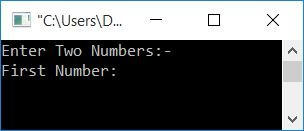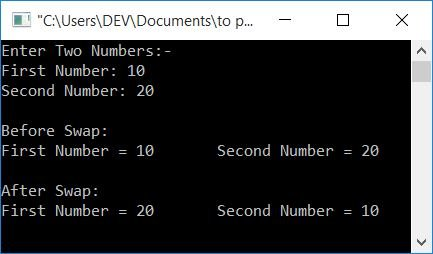# C Program to Swap Two Numbers

In this article, you will learn how to code the swapping of two numbers in the C language using the following approaches:

### What is "Swapping of Two Numbers"?

When two numbers are swapped, the first becomes the second and the second becomes the first. For example, if the user enters any two numbers, say 10 and 20. And let's suppose the two variables, say, num1 and num2, hold these two numbers. That is, num1 = 10 and num2 = 20. Then, after swapping, it will be like num1 = 20 and num2 = 10.

## Swap two numbers using a third variable in C

The swapping of two numbers in C becomes easy using the third variable. That is, this program uses a variable named "temp" that helps a lot while performing the swap operation of two numbers given by the user at run-time. Let's take a look at the program first.

```#include<stdio.h>
#include<conio.h>
int main()
{
int num1, num2, temp;
printf("Enter Two Numbers:-\n");
printf("First Number: ");
scanf("%d", &num1);
printf("Second Number: ");
scanf("%d", &num2);
printf("\nBefore Swap:\n");
printf("First Number = %d\tSecond Number = %d", num1, num2);
temp = num1;
num1 = num2;
num2 = temp;
printf("\n\nAfter Swap:\n");
printf("First Number = %d\tSecond Number = %d", num1, num2);
getch();
return 0;
}```

This program was built and runs in the Code::Blocks IDE. Here is its sample run:Now enter the first number, say 10, and then the second number, say 20. Press the ENTER key to see the following output:The main block of code for swapping two numbers is:

```temp = num1;
num1 = num2;
num2 = temp;```

For example, if the user enters 10 as the first number, then 10 gets initialized to num1. And if the user enters 20 as a second number, then 20 gets initialized to num2. That is, num1 = 10 and num2 = 20.

Now, after executing the statement:

`temp = num1;`

The value of num1 (that is, 10) gets initialized to temp. Therefore, temp=10. And after executing the statement,

`num1 = num2;`

The value of num2 (that is, 20) gets initialized to num1. Therefore, num1=20. And again after executing the statement:

`num2 = temp;`

The value of temp (that is, 10) gets initialized to num2. Therefore, num2=10. As a result, the variable num1 now has the same value as num2. Whereas the variable num2 holds the initial value of num1.

Note: Don't forget to initialize the value of num1 to any third variable before initializing the value of num2 to it.

## Swap two numbers without using a third variable in C

Unlike the previous program, this program will not use any type of extra (third) variable in either a direct or indirect way.

```#include<stdio.h>
#include<conio.h>
int main()
{
int num1, num2;
printf("Enter Two Numbers:-\n");
printf("First Number: ");
scanf("%d", &num1);
printf("Second Number: ");
scanf("%d", &num2);
printf("\nBefore Swap:\n");
printf("First Number = %d\tSecond Number = %d", num1, num2);
num1 = num1+num2;
num2 = num1-num2;
num1 = num1-num2;
printf("\n\nAfter Swap:\n");
printf("First Number = %d\tSecond Number = %d", num1, num2);
getch();
return 0;
}```

This program will produce the same output as the previous one. The following block of code is responsible for swapping two given numbers. Here I've used the addition and subtraction operations:

```num1 = num1+num2;
num2 = num1-num2;
num1 = num1-num2;```

For example, let's suppose 10 and 20 are the two numbers entered by the user. Therefore. num1 equals 10 and num2 equals 20. Now, after executing the following statement:

`num1 = num1+num2;`

The value comes from num1+num2 (that will be 10+20) and gets initialized to num1. Therefore, num1=30. Again, after executing the following statement:

`num2 = num1-num2;`

The value comes from num1-num2 (that will be 30-20) and gets initialized to num2. Therefore, num2=10. And again, after executing the statement given below:

`num1 = num1-num2;`

The value comes from num1-num2 (that will be 30-10) and gets initialized to num1. Therefore, num1=20. The value of the first variable is now transferred to the second, and the value of the second variable is transferred to the first.

### The Swapping Concept of Using + and -

If there are two numbers. Assume the first number is the total.Then, if you subtract the second number from the total, you'll get the first number. Therefore, it gets initialized to num2 (which now holds the initial value of num1). Again, if you subtract the new value of the second number (the initial value of the first number) from the total, you will get the initial value of the second number. Therefore, it gets initialized to num1 (which now holds the initial value of num2).

## Using a Function and a Pointer, swap two numbers in C

This is the last program on the swapping of two numbers. This program uses a user-defined function named swapFun() to swap the two numbers given by the user:

```#include<stdio.h>
#include<conio.h>
void swapFun(int *, int *);
int main()
{
int num1, num2;
printf("Enter Two Numbers:-\n");
printf("First Number: ");
scanf("%d", &num1);
printf("Second Number: ");
scanf("%d", &num2);
printf("\nBefore Swap:\n");
printf("First Number = %d\tSecond Number = %d", num1, num2);
swapFun(&num1, &num2);
printf("\n\nAfter Swap:\n");
printf("First Number = %d\tSecond Number = %d", num1, num2);
getch();
return 0;
}
void swapFun(int *num1, int *num2)
{
int temp;
temp = *num1;
*num1 = *num2;
*num2 = temp;
}```

This program also produces the same output as the previous two programs.

In the above program, the & is called the address of the operator, whereas the * is called a value at the operator. Therefore, using the address of operator, we've passed the address of both the variables, say, num1 and num2 (that holds the two numbers entered by the user), to the function named swapFun().

And inside the function swapFun(), we have used the value at operator to fetch the value available at the addresses of both variables. The two variables get swapped inside the function. Because we're using the addresses of both variables, the operation that has been done inside the function swapFun() affects the variable's value throughout the program. That is, when we print the value of two variables inside the main() function (after calling the function swapFun()), their value get swapped.

#### The same program in different languages

C Quiz

« Previous Program Next Program »